## Wednesday, June 18, 2008

### Make Complex Numbers Real

This post is continuation of this rant.

We do such a bad job with complex numbers that students often assume that the word Imaginary in front of Number is an adjective that i is not really a number. It is some sort of imaginary thing.

One important reason that students find this easy to believe is the fact that it is not possible to order the complex numbers. Ordering is a basic and fundamental concept for numbers. Ordering is first thing that students are to learn about numbers. We stress the idea of ordering of the numbers, we use the ordering of numbers to help explain addition and subtraction. So if we can neither tell if i is neither bigger or smaller than 0, then it might not really exist.

So makes imaginary and complex numbers real. What makes them real is that can represent things that happen in the real world. Real numbers can represent length and direction (direction in one dimension, left or right, up or down). Complex numbers can represent length and direction in two dimensions. So 3+4i can represent 3 steps to the right and 4 steps up. You can represent movement in two dimensions with complex numbers. But that is not different than vectors. What makes complex numbers so different is the existence of a coherent multiplication rule, one that even allows a coherent definition of division.

So it is multiplication that makes complex numbers so special. What does multiplication in complex numbers do. It does two things, it is capable of doing what multiplication of real numbers does, which is dilation. Multiplication by a positive real number performs a dilation on the points of the complex plane. Multiplication by a negative real number performs a dilation and a 180° rotation. In particular, multiplication by -1 is just a 180° rotation. Now how about multiplication by i. If you take any complex number and multiply it by i the result is a 90° rotation about 0. And from all that we know about i, this makes tremendous sense. We know that i is the square root of negative one, so that multiplying by i does half of what multiplying by -1 does. We know that i^4=1 so that multiplying by i four times does nothing to a number, just as rotating by 90° four times returns things to where they started. It turns out then that multiplying by complex numbers models rotation! This is what is important. Turns are everywhere. Everywhere in life there are rotations of all sorts and these numbers allow you to do computations with coordinates that affect the desired rotations. Want to turn 90°, multiply by i. Want to turn 45°, multiply by sqrt(i). This is why polar form for a complex number exists, and it is the importance of DeMoivre's Theorem.

As a final note tonight. Let's bring it back to algebra. Let's say we have to solve x^6=1. Then we are looking for 6 solutions. Well then these are complex numbers that multiplication is the same as rotating 0°, 60°, 120°, 180°, 240°, and 300°. In other words 1, 1/2 +sqrt(3)/2 i, -1/2 +sqrt(3)/2 i, -1, -1/2 -sqrt(3)/2 i, 1/2 -sqrt(3)/2 i. But what is better is seeing it on the complex plane.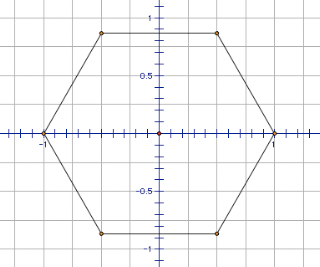That is right the solutions to this equation are vertices of regular polygon, and more this generalizes. So that the regular polygons can be associated to the polynomials x^n-1.

## Tuesday, June 17, 2008

### Why do we make it so complex???

Are you a math teacher? If you are then you probably have built up a tolerance to the funny looks; a tolerance to the subtle comments that are meant to infer that you are VERY different from everyone else. You must have this tolerance or how else could you continue in your profession. I understand. I have it too.

But here is the thing. It dulls us to the messages around us. Something is wrong in in the state of math education. Most of us know this, though some still fight it. Most of us know that it is the charge of math teachers to make it better. We must raise an entire generation of children that meet math teachers and say, "Yeah, math was always too easy for me so I decided to do something difficult like teach grammar instead." That is our charge, and to make it happen we need to assiduously evaluate everything that we do. We must find the mistakes and fix them. I don't know if what I am about to propose a solution to is the most pressing problem, but it definitely is one. We may be able to learn something from the problem, and I hope something from my proposed solution. Here goes...

Complex Numbers

Let's take what a child is supposed to know about complex numbers after Algebra 2. Algebra 2 is or was a terminating course in high school math. So this may be all that any one every learns about these crazy things. Here are the objectives from the Algebra 2 book I am teaching out of during summer school:

• multiply pure imaginary numbers
• solve quadratic equations that have pure imaginary solutions
• add, subtract, and multiply complex numbers
• simplify rational expressions containing complex numbers in the denominator
Wow. How incomplete a person would feel if they had not accomplished these feats of learning.

This is really the first time that students have been exposed to these kinds of numbers. Some have heard about them and wondered what they meant. And what do get to find out about them. That the purpose of complex numbers is to be added, multiplied, subtracted and simplified. Imagine that you were trying to sell and innumerate person on the integers.

Stone Age English Major: Cool what can you do with it?

Stone Age Math Teacher: You can add, subtract, multiply and divide with it. Pretty cool huh? And you can solve any subtraction problem if you allow a whole class of new numbers called the integers!

Stone Age English Major: Wow. Major. Let's go invent beer, so I can invent poetry.

A purpose for complex numbers must be the ground work to any introduction to these numbers. Many of you may feel that these numbers have no purpose. Or that maybe the purpose is to allow us to say that yes, every quadratic does have two solutions (if you count multiplicities). But no, the purpose is real. It is part of our everyday existence. Just as the reason that 1, 2, 3 came about was to help sheep herders keep track of the flock. We are talking real.

What is it. Yawn.... I will write that tomorrow.

## Sunday, June 15, 2008

### Cat's (Synthesized) Meow!

Our latest post on determinants made the carnival of mathematics at catsynth.com. Which reminds me of one of the things that makes me happy in the world... Cats falling compilation videos on youtube. One of my AP Calc BC kids showed me this one. Those kids are so smart.

I really love the music. I always wondered what the purpose "Man in motion" was.

## Friday, June 13, 2008

### Discriminating determinant

So far we have found that we can use the determinant to find area and volume, and that they can also help us reform the concept of proportion. Determinants can provide a way to calculate this quantity in some cases where other methods could be much more difficult. We have seen that the determinant is a generalization of proportion. What more?

One of the most important properties of determinants is that if two rows (or columns) are the same then the determinant is zero. This can be easily seen from the area interpretation of the determinant, because if two columns are the same then the area defined is degenerate for that dimension. The box has been flattened.

This is very similar to another way in which we discriminate things in math. In algebra we often see the quantity (x-a). This comes up in factoring or even in creating polynomials that have specific quantities, such as Lagrange Interpolation.

Using determinants to discriminate

Just like the expression (x-a) has the ability to test if the value of the variable x is equal to a, Discriminant will allow us to test if many variables have desired quantities simultaneously.

Example: Finding the equation of a line

In this example uses the multi-linearity of the determinant to find the equation of a line through two points. For example let’s try to find an equation of a line through (3, 5) and (-2, 3). To do this with determinants we set up the following 3x3 determinant equation.Clearly, the points (3, 5) and (-2, 3) satisfy this equation, so that solution set does go through those two points. Is it a line? Well from any method that you choose to evaluate the determinant you can see that the coefficient of x and y will be numbers. Therefore it is a line.

Why are the in the third column? Well we need to make sure that we have a square matrix to take the determinant of, and we need to make sure that the three rows have the same number. So that we can get the determinant to evaluate to zero when we insert the points we know.

Example: Equations for other curves

One problem that we often ask students in Algebra 2 is to find the equation through three points. Let’s do one now, for instance find an equation through the points (3, 5), (-2, 3), and (6, 9). Then we set up the following determinant equation.The numbers in the first column are the squares of the numbers in the second column. In fact, we can find an equation for a circle through those three points by the following equation.Conclusion

Determinants may have more use and meaning that we have given them credit for in the standard high school curriculum. Can we bring them to the students in a meaningful way? Can we show them their usefulness and their beauty? I encourage you to explore this with students, friends

## Thursday, May 29, 2008

### Schlope and PMAN

Mathematics is the art of giving the same name to different things. - Henri Poincare
So in this case it is not the quite the same name, but I have sort of "invented" some terminology. The idea of "schlope". Schlope is quite similar to slope. The slope of a line tells you how much to go up for every 1 that you move to the right. Schlope tells you what to multiply by for every one step to the right. Schlope is very important for exponential functions. Generally, in exponential situations we talk about the "rate of growth". If the rate of growth is 8% then the function that models that growth has "1.08 to the t" in it. That 1.08 is what I call "schlope". So, schlope is the number that you actually use in the formula.

In fact, you can create parallels for all your favorite formulas and constructs from linear functions. This parallel construction highlights another construct of mine that I like to call the hierarchy. The hierarchy is simply the recognition that the fundamental ways of putting numbers together come in an order. At the bottom level we have Addition and Subtraction. In the middle we have Multiplication and Division, and at the top powers, roots, and logarithms. Another teacher at my school refers to this as "PMAN" for powers, multiply, add, nothing. It is very helpful to think about PMAN when doing calculations with exponents and logs. If you are taking powers of powers, "P", then you "M"ultiply the powers. If you are "M"ultiplying powers with the same base then your "A"dd the exponents. If you are "A"dding different powers of the same base together then you do "N"othing.To move from Linear functions to Exponential functions you simply must move up in the hierarchy. Moving from repeatedly adding a number to the height every time to multiplying that height by some fixed number. The formulas can be written quite similarly:### Highlights Highlight

My wife and I wrote an article in Highlights magazine about factorial. You probably know the magazine from the doctors office. You can read the article here Big numbers at a banana-split bar but you miss all the pictures. Guess you will have to find it at the dentists office.

## Wednesday, May 28, 2008

### Are quadratics really that important

If I was a student who had just finished a course in Algebra I in most schools across America I think that I would think that the following were the most important things that we had done. I would think this because we had spent so much of our efforts on it.

• linear equations
• distributive law
• factoring
The problem with this list isn't so much what is on it, it is what isn't on it. It seems to me that we simply must include exponential functions and therefore also logarithms. I guess my question is, "Are quadratics really that important?"

The only way to add these topics in is to take other topics away. So what can go? Here is a partial list of topics that might be cut or reduced in the standard curriculum.

• completing the square
• conics
• rational root theorem
• long division of polynomials
Exponentials seem like such a natural topic, and one that could be made to support and build on linear equations so beautifully. I will give more of this idea when I write later about "schlope".

## Sunday, May 25, 2008

### Determinants as proportions

This is a continuation of a discussion of determinants started here. And there is more here.

Proportions

So if determinants are important how important are they and when can they be introduced reasonably. One place that they could possibly come up is in reference to proportions. As a reminder, a proportion is equation where two ratios are equal. One method to solve equations such as this is the Means-Extremes Property. This is more commonly known as “Cross-Multiplying”. I think I speak for many teachers that cross-multiplying is a “bane of existence”. Cross-multiplying is a rule that is often overused. It seems to quickly rise to the top of all students list of favorite methods so that whenever in doubt about how to proceed in a problem with fractions teachers often here the idea put forth that the correct method might include cross-multiplying. This is probably because few students really understand what this method does or why it works

Connections between determinants and proportions

So what is the connection between determinants and cross multiplying. Well
it can be seen from a variety of ways. First is in the formulas themselves. A
proportion has the form: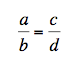after cross-multiplying we know thatthis last equation can be rewritten in terms of determinants asIn a proportion we are given that two fractions are the same. Each of those fractions can be thought of as vectors. Similar to the definition of slope as a fraction or as a vector. With this definition of the fraction we see that the two fractions will be equivalent if their vectors point in the same direction. If they point in the same direction then the area given by the determinant will be zero.

The advantage of this determinant method to solving proportions is that we eliminate the fractions from the problem. Cross-multiplying would only exist in determinants, where the rightly do play a role. Students would be less likely to misapply the idea of cross-multiplication to every situation with fractions. Determinants would be introduced earlier and their presentation of area would be well supported. Students would also have to have a clearer understanding of slope as a primary way of looking at fractions.

## Wednesday, May 14, 2008

### What is a determinant?

Determinants pros/cons
Determinants, for a teacher if I may borrow a British phrase, they are a bit of a sticky wicket. For my teaching, determinants have the qualities that I find makes a topic uninviting for students:

➢ The rules for manually calculating determinants seem arbitrary, and are nearly impossible to motivate.
➢ It is not clear what the determinant means. By which I mean it is not clear what “natural” meaning might be given to a the value of a determinant, in much the same way that slope can be imbued with the natural meaning of “rate of change”.

But determinants are also tempting:

➢ Cramer’s rule presents a powerful way to solve systems of equations
➢ Matrices are a vast generalization of number systems that have far reaching applications. Can we use determinants as a way to motivate the study of these objects?

When teaching we need motivations
The first question I ask about a topic, is what might lead someone to want to know this? Why have “slope” or a “determinant”. For motivation, slope benefits from the universality of change. A recurrent theme in mathematics education from algebra to calculus is the wide range of applications of the slope concept to measure change.
Each child must see the motivation in mathematics
History of math as a motivation
What can we use to motivate determinants? Often when I find myself struggling to motivate something I will study the historical motivations of a topic. For instance, the rules of probability are often well motivated by the story of the way Fermat and Pascal were lead to discuss a game that motivated many questions about probability.
The determinant’s history may not be as storied. It begins to late in the history of mathematics to have a wonderful story attached. Professional mathematicians, not interested lay practitioners brought it forth.
Connections/Generalizations of previous curriculum
To be a topic worthy of our student’s interest it must be made to represent something meaningful in their lives, in their experience, or in the larger life of the mind. Can we at least show that a curious soul would want to see what this is all about? We can only do this if the determinant can tell us something that our students find real. Rules that utilize determinants in part, such as Cramer’s rule, are inadequate for this job because the determinant is only part of the equation. Why not make the whole thing one giant new equation? We need to start with unadorned determinants to begin with.

In the rest of this article I will try to make the leap. I will try to motivate determinants and demystify them, and I will indicate some more uses for them. The more we understand about the determinants the more our students will understand them and find even more remarkable ways to use them.

Volume and Area
Volume and Area do provide a very concrete motivation. It is clear to a student that measuring the size of objects is a fundamental question of existence. There are two formulas for area that I would like to focus on. These are the standard the equations for the area of a parallelogram and the area of a triangle.

The inadequacies of some formulas for some area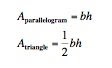These formulas are absolutely correct and deeply flawed. The major drawback of these formulas is that they rely on a length that is not truly “part” of the shape. The height measurement would in reality be a difficult measurement to determine. How is it possible to determine that we are really are measuring an altitude?

Determinants provide another method to find area. In this version we must assume that the vertices have been given coordinates. Then it is possible to find vectors that represent the sides. These vectors not only encapsulate the length of the side but also the direction. It is therefore possible to find the area of a parallelogram or triangle from these measurements.

For instance, take the parallelogram shown below.In this case we can compute the vector from A to B is <> and from A to D is <>. Using these vectors we can compute the determinant and from that the area.Because we are interested in computing with vectors we can in general imagine that one of the corners of the parallelogram is at the origin. This gives us a figure like the one below.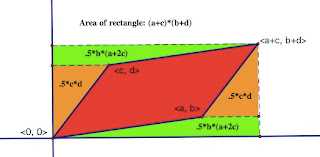We can then compute the area of the parallelogram by finding the area of the rectangle and then subtracting the area of the green trapezoids and orange triangles.This shows that the area of the parallelogram can be computed from the vectors that define the parallelogram. Because triangles are half the size of a parallelogram we can compute the area of triangles similarly.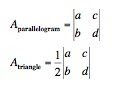Where and are the vectors that define two adjacent sides of our shapes.

This analysis can be extended to parallelepiped in three dimensions.

Next, we look at applying the determinant to problems involving proportions.

## Monday, February 18, 2008

### A tease

I keep forgetting about all the things I want to write about. So I will make a public list and hopefully these posts will come in quick succession.

• Tchebyshev Polynomials (Thanks to Al Cuoco again)
• Combinatorial Game Theory textbook
• Blogging as an assignment
• The genius of Euler
Feel free to write in the comments that one of these is more interesting than the next.

### More Determinants

I was at the Joint Mathematics Meeting in January, and their I met an author that I really enjoy. His name is Al Cuoco. He does a lot with mathematics education and his book on Mathematical Connections is a must for any high school math teacher who wants to give their students a hint of the beauty of mathematics beyond high school math. I highly recommend it.

Since he was there, and he was speaking a little bit about linear algebra, I decided to ask him the question that has been bothering me for at least a year. What is the the determinant? Remember from my previous posts that it is not that I don't know how to calculate a determinant, or when to use a determinant. What I don't know is how to explain it to students. How to give it meaning. Al's opinion appears to be that it is most clearly connected to volume. To help prove his point he sent me a graphic which previous appeared on the cover of a math journal. The graphic does a pretty good job of explaining how determinants can be used to find the coordinates in a different coordinate system. It basically comes down to projections.

Pretty cool. I think that I will try to tie together the different determinant thoughts that I have had over the year in one post. I will hopefully be able to add some of the things that I have learned in Al's papers.

## Thursday, February 14, 2008

### Calculator programming

While covering the quadratic formula with my 8th grade algebra students, I decided to show them how to program their calculator to do the quadratic formula. Students had a surprisingly strong response to it. They loved it.

Certainly they love the power of having the quadratic formula in their calculator. And their may be less chance that they will memorize the quadratic formula because they don't have to do it as often as they would have had to if I hadn't given them the formula. But it was a great way to discuss the ideas of input and output. I drew a function machine on the board, and we discussed how other machines can be seen as functions. So this connects this fundamental mathematical concept of function for them to their lives, and it allows them a way to construct a function of their own. We did a problem of finding how long it takes for an object to fall a certain distance. Students really started to get it.

In fact, after we programmed that we wrote a program to find the vertex, both the x and y coordinates. This was great for them because it emphasized that this process of writing a computer (calculator) program is really whatever you want. Got a process that you are doing over and over then make a program. I also indicated that these two separate programs might be merged together to create a bigger more complex program that could do both things. Other teachers told me later that the students were still talking about it several periods later.

On the technology side, it really helps to do this with a smartboard and TI-smartview. I was asked so rarely about what did I type or where that button is on the calculator. It is such a relief to have these tools.

## Sunday, February 10, 2008

### Bear hunting Algebra

I am finding myself more of a shameless shill for mathematics in my 8th grade Algebra class. Want me to jump around like an idiot? I will do it. Want me to make a snowball and bring it in to the classroom? Good as done. So you can see I am interested doing anything that will meet my students where they are...

One more crazy thing I have been doing is trying to connect the kids books I read to my math class. And I think I found out one that actually worked a little bit. It is Going on a Bear Hunt...

You may the know story, you may have been to camp, you may have never heard of it, which may mean that you have never had a child, been to any place where teenagers are asked to supervise young children. If that is you click here to get a sense of what it means to go on a bear hunt.

I use this to remind/reinforce the idea about doing and undoing to solve an algebraic equation. So with the equation:

3x+1=10

I say we are going on bear hunt we are going catch a big one we are not scared. Oh no a times 3!. Can't go over it can't go under it... Oh no a plus 1, etc. And then I said a 10! AAAAAAHHHHHH! Back through the 1 (minus 1), back through the 3 (divide by three). These are the steps (minus 1 and divide by 3) that you need to solve the problem.

Now as many of surely know, much of this is not "necessary", but my students need the background of reading through the problem the first time to understand the order of operations. In fact, many of my students still don't understand that the 3x cannot be undone by subtracting 3.

I hope that this helps them see not only what to do, but why they are doing it.

At the very least I am exposing them to classic literature, well literature, well words.